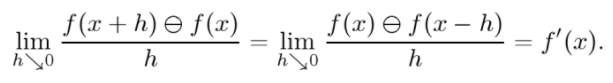# Hukuhara Derivative: Definition

Derivatives > Hukuhara derivative

Generalized Hukuhara differentiability is the most general type of differentiability for interval-valued functions . Hukuhara introduced the idea of the Hukahara derivative (H-Derivative) in his 1967 paper , which became a starting point for the study of set differential equations and fuzzy differential equations .

## Hukuhara Derivative: Definition

Hukuhara first described the Hukahara derivative of f at x as the fuzzy number (an imprecise number) f′(x), defined as:

A function f: (a, b) → ℝF is Hukahara differentiable if, for sufficiently small h > 0, the H-differences
f (x + h) ⊖ f(x) and f(x) ⊖ f(x – h) exist and if there is also an element f′(x) ∈ ℝF such that :Hukuhara defined the “H-difference” to overcome the problem of finding a suitable difference between two intervals for interval-valued functions:

• If A = B + C, then the H-difference is A and B (denoted by A – HB) equals C.
• For arbitrary pairs of intervals, the H-difference may or may not exist .

## Generalized Hukuhara Derivative

The “classical” Hukuhara derivative from Hukuhara’s paper has a shortcoming: solutions for interval differential equations (IDEs), which are interval-valued mappings, have values with non-decreasing length. This means that the uncertainty shown by the solutions grows over time . This “new” version (the generalized Hukuhara derivative) removed the problem of these time-sensitive, imprecise solutions.

Stefanini and Bede’s  research on interval differential equations introduced a new version of the Hukuhara derivative for interval-valued mappings. Their approach has solutions where values have non-increasing length.

## The H-Difference

Hukuhara defined a new difference for compact and convex sets. The Hukuhara difference (H-difference), a special case of the Minkowski difference, is one way to handle the inverse element problem for compact and convex sets. The inverse element drawback describes how convex sets have a linear structure with respect to Minkowski sum and scalar multiplication. This structure is a cone instead of a vector, because the inverse element of a set (with respect to the Minkowski sum) may not exist. To put this another way, if A = {a} isn’t a singleton set, the Minkowski sum of A and −A may not be the identity element {0}—. In other words, A + (−1)A ≠ {0}. Hukuhara’s studies also led to the development of the H-difference of fuzzy sets and fuzzy number-valued functions..

## References

 Cano, Y. et al. (2012). Calculus for interval-valued functions using generalized Hukuhara derivative and applications. Retrieved November 23, 2021 from: https://www.sciencedirect.com/science/article/abs/pii/S016501141200509X
 M. Hukuhara, Intégration des applications mesurables dont la valeur est un compact convexe, Funkcial. Ekvac. 10 (1967) 205–223.
 Cano, Y. et al. Calculus for interval-valued functions using generalized Hukuhara
derivative and applications. Fuzzy Sets and Systems 219 (2013) 49–67.
 Bede, B. (2012). Mathematics of Fuzzy Sets and Fuzzy Logic. Springer Berlin Heidelberg.
 Malinowski, M. Interval differential equations with a second type Hukuhara derivative. Retrieved November 23, 2021 from: https://core.ac.uk/download/pdf/82239432.pdf
 L. Stefanini, B. Bede, Generalized Hukuhara differentiability of interval-valued functions and interval differential equations, Nonlinear Anal. 71 (2009)
1311–1328.
 Okin, A. & Bayeg, S.The concept of Hukuhara derivative and Aumann integral for intuitionistic fuzzy number valued functions. MANAS Journal of Engineering MJEN Volume 6 (Issue 2) (2018) Pages 143-163.

CITE THIS AS:
Stephanie Glen. "Hukuhara Derivative: Definition" From StatisticsHowTo.com: Elementary Statistics for the rest of us! https://www.statisticshowto.com/hukuhara-derivative/